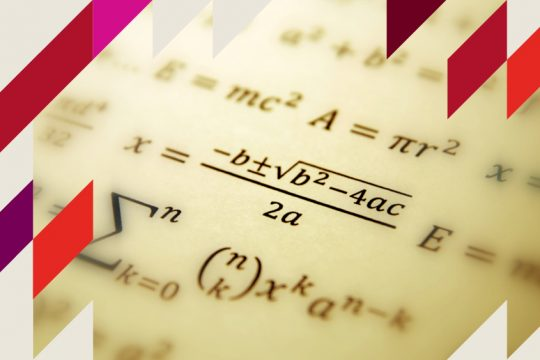# B.Sc Mathematics

It is s an Undergraduate Mathematics course. Mathematics is the study of quantity, structure, space and change. Mathematicians seek out patterns and formulate new conjunctures. This degree course is very important and career orienting.

Core Courses

• Foundations of Mathematics
• Calculus
• Calculus and Analytic Geometry
• Theory of Equations
• Matrices and Vector Calculus
• Vector Calculus
• Abstract Algebra
• Basic Mathematical Analysis
• Differential Equations
• Real Analysis
• Complex Analysis
• Numerical Methods
• Number Theory and Linear Algebra

Complementory Courses

• Basic Statistics and Probability
• Probability Distributions
• Statistical Inference
• Applied Statistics
• Computer Fundamentals
• Programming in C
• Fundamentals of System
• Software,Networks & DBMS
• Visual Programming
• Programming Lab: C & Visual Programming#### Course Plan

##### Affiliation
• University of Calicut
• Mathematics
##### Eligibility
• The basic eligibility criteria is qualifying 10+2 or equivalent examination in any stream.
• B.Sc
##### Duration
• Six Semesters
##### Admission Procedure
• No Entrance Examination: Admission on merit basis.
• Full Time
Green Campus